# Test: Tangents to a Circle

## 25 Questions MCQ Test Online MCQ Tests for Class 10 | Test: Tangents to a Circle

Description
Attempt Test: Tangents to a Circle | 25 questions in 25 minutes | Mock test for Class 10 preparation | Free important questions MCQ to study Online MCQ Tests for Class 10 for Class 10 Exam | Download free PDF with solutions
QUESTION: 1

Solution:
QUESTION: 2

### The length of the tangent drawn from a point 8 cm away from the centre of a circle, of radius 6 cm, is :​

Solution:

Since tangent is perpendicular to radius, the triangle so formed is a right angled triangle,
So using Pythagoras Theorem,
Line joining centre and And the point outside the circle is hypotenuse and tangent and radius are the two sides
H2=P2+B2
64=P2+36
P=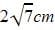QUESTION: 3

### Number of tangents, that can be drawn to a circle, parallel to a given chord is

Solution:

There are only two tangents that can be drawn parallel to a given chord. That is the tangents are drawn on either side of the chord so that both are parallel to the chord.

QUESTION: 4

How many tangents can be drawn to a circle from a point in its interior?​

Solution:
QUESTION: 5

The tangents drawn at the ends of a diameter of a circle are:​

Solution:
QUESTION: 6

A circle can pass through​

Solution:

The answer can be 2 collinear points as well. We have three collinear points. Join one point to the other two points and then draw the perpendicular bisector for both the lines
Join the perpendicular bisectors. The point is the centre of the circle. And a circle can be formed then. Also two points can form a circle as the two points joines becomes a diameter.

Option D : The number of circles which can pass through three given non-collinear points is exactly one.

So, A is the correct Option

QUESTION: 7

In the given figure, PA and PB are tangents from P to a circle with centre O. If ∠AOB = 130°, then find ∠APB.

Solution:

In AOB, by angle sum property
Angle A + Angle B + Angle O=180°
2Angle A = 50°       (Triangle is isosceles triangle)
Angle A = 25°
We know Angle APB= 2Angle A
Angle APB=2*25= 50°

QUESTION: 8

From a point A, the length of a tangent to a circle is 8cm and distance of A from the circle is 10cm. The length of the diameter of the circle is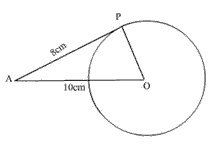Solution:
QUESTION: 9

PQ is a tangent drawn from a point P to a circle with centre O and QOR is a diameter of the circle such that ∠POR =120o, then find ∠OPQ.

Solution:
QUESTION: 10

If figure 1, O is the centre of a circle, PQ is a chord and PT is the tangent at P. If ∠POQ = 70o, then ∠TPQ is equal to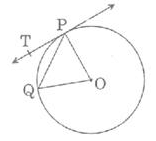Solution:

POQ is an isosceles triangle because of 2 radii as sides. So by angle sum property, 2*angle OPQ=180-70=110
Angle OPQ=55°
Since Angle TPO is a right angle , because PT is a tangent,
Angle OPQ+Angle TPQ=90
Angle TPQ=90° - 55° = 35°

QUESTION: 11

A line that intersects a circle in exactly one point is called a​

Solution:
QUESTION: 12

A line that intersects a circle in two distinct points is called a

Solution:

A chord is the actual line segment determined by these two points, that is, the interval on the secant whose ends are at these positions But a secant of a curve is a line that intersects the curve at a minimum of two distinct points. The difference is that the secant is not just limited to the circle it extends further.

QUESTION: 13

The length of tangent drawn from an external point P to a circle, with centre O, is 8 cm. If the radius of the circle is 6 cm, then the length of OP (in cm) is:​

Solution:
QUESTION: 14

A line segment having its end points on the circle is called a​

Solution:
QUESTION: 15

Number of tangents from a point lying inside the circle is​

Solution:
QUESTION: 16

The angle between two tangents drawn from an external point to a circle is 110°. The angle subtended at the centre by the segments joining the points of contact to the centre of circle is:

Solution:
QUESTION: 17

A tangent PA is drawn from an external point P to a circle of radius 3√2 cm such that the distance of the point P from O is 6 cm as shown figure. The value of ∠APO is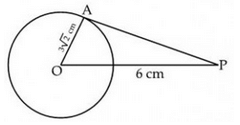Solution:

In right angled triangle,  OAP

sin of angle APO = AO/OP = 1/root2

sin 45 = 1/root2

angle APO = 45 degree

QUESTION: 18

What is the distance between two parallel tangents of a circle of the radius 4 cm?​

Solution:
QUESTION: 19

In fig., two concentric circles of radii a and b (a > b) are given. The chord AB of larger circle touches the smaller circle at C. The length of AB is: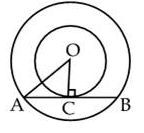Solution:
QUESTION: 20

A tangent to a circle is a line that intersects the circle in

Solution:
QUESTION: 21

In the figure, if from an external point T, TP and TQ are two tangents to a circle with centre O so that POQ = 110°, then, PTQ is: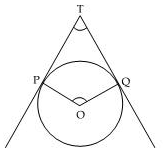Solution:
QUESTION: 22

In fig., two circles with centres A and B touch each other externally at k. The length of PQ (in cm) is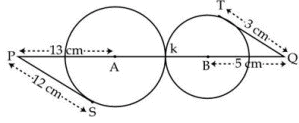Solution:
QUESTION: 23

The distance between two parallel tangents to a circle of radius 5 cm is​

Solution:
QUESTION: 24

A circle may have…….​

Solution:
QUESTION: 25

In fig., PA is a tangent to a circle of radius 6 cm and PA = 8 cm, then length of PB is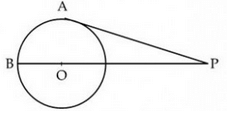Solution:Use Code STAYHOME200 and get INR 200 additional OFF Use Coupon Code